• package tao.leetcode; import java.util.*; /** * Created by Tao on 2017/7/30. */ public class ...//第列由大到小排列 return b[ 0 ] - a[ 0 ]; /// /第一列由大到小排列 } }
package tao.leetcode;
import java.util.*;
/**
* Created by Tao on 2017/7/30.
*/
public class MyLeetcode {
public static void main(String[] args) {
int[][] people = {{7,0}, {4,4}, {7,1}, {5,0}, {6,1}, {5,2}};
final int[] arOrders = {1,0};
Arrays.sort(people, new Comparator<Object>() {
public int compare(Object oObjectA, Object oObjectB) {
int[] arTempOne = (int[])oObjectA;
int[] arTempTwo = (int[])oObjectB;
for (int i = 0; i < arOrders.length; i++) {
int k = arOrders[i];
if (arTempOne[k] > arTempTwo[k]) {
return 1;
}
else if (arTempOne[k] < arTempTwo[k]) {
return -1;
}
else {
continue;
}
}
return 0;
}
});

for (int i = 0; i < people.length; i++) {
for (int j = 0; j < people.length; j++) {
System.out.print(people[i][j] + " ");
}
System.out.println();
}
}
}

output：  5 0  7 0  6 1  7 1  5 2  4 4  其中arOrders[]控制排序列的顺序
        Arrays.sort(people, new Comparator<int[]>() {
public int compare(int[] a, int[] b) {
if (b == a) return a - b; //第二列由大到小排列
return b - a; ////第一列由大到小排列
}
}
展开全文排序
• VB.NET二维数组快速排序（更新） 'OldArrays(),为排序二维数组;NewArrays(),为存放结果数组,SortColumnsOrOrders()，传递排序参数数组,偶数个为排序列号，奇数为升降序，0为升序，1为降序；FieldRow，是否有字段行...
• 今天在做下面这道题的时候糊涂了，把二维数组的排序做错了。 题目 假设有打乱顺序的一群人站成一个队列，数组 people 表示队列中一些人的属性（不一定按顺序）。每个 people[i] = [hi, ki] 表示第 i 个人的身高为 ...
点击查看更多通信与专业知识
今天在做下面这道题的时候糊涂了，把二维数组的排序做错了。
题目
假设有打乱顺序的一群人站成一个队列，数组 people 表示队列中一些人的属性（不一定按顺序）。每个 people[i] = [hi, ki] 表示第 i 个人的身高为 hi ，前面 正好 有 ki 个身高大于或等于 hi 的人。
请你重新构造并返回输入数组 people 所表示的队列。返回的队列应该格式化为数组 queue ，其中 queue[j] = [hj, kj] 是队列中第 j 个人的属性（queue 是排在队列前面的人）。
示例 1：
输入：people = [[7,0],[4,4],[7,1],[5,0],[6,1],[5,2]] 输出：[[5,0],[7,0],[5,2],[6,1],[4,4],[7,1]] 解释： 编号为 0 的人身高为 5 ，没有身高更高或者相同的人排在他前面。 编号为 1 的人身高为 7 ，没有身高更高或者相同的人排在他前面。 编号为 2 的人身高为 5 ，有 2 个身高更高或者相同的人排在他前面，即编号为 0 和 1 的人。 编号为 3 的人身高为 6 ，有 1 个身高更高或者相同的人排在他前面，即编号为 1 的人。 编号为 4 的人身高为 4 ，有 4 个身高更高或者相同的人排在他前面，即编号为 0、1、2、3 的人。 编号为 5 的人身高为 7 ，有 1 个身高更高或者相同的人排在他前面，即编号为 1 的人。 因此 [[5,0],[7,0],[5,2],[6,1],[4,4],[7,1]] 是重新构造后的队列。 示例 2：
输入：people = [[6,0],[5,0],[4,0],[3,2],[2,2],[1,4]] 输出：[[4,0],[5,0],[2,2],[3,2],[1,4],[6,0]]
提示：
1 <= people.length <= 2000 0 <= hi <= 106 0 <= ki < people.length 题目数据确保队列可以被重建
来源：力扣（LeetCode） 链接：https://leetcode-cn.com/problems/queue-reconstruction-by-height
C++代码 解法
思路：

//因为第二个数表示的是前面有多少个人大于等于他的身高 所以身高越高的受限制越少 则先把身高比较矮的安排了即可         //根据身高升序排序 同时个数降序排序(受限制多的先排)         //使用一个数组记录未安排的位置 每安排一个就删掉一个         // 示例：         // [ 0, 1, 2, 3, 4, 5 ] [ 4, 4 ] 4         // [ 0, 1, 2, 3, 5 ]    [ 5, 2 ] 2         // [ 0, 1, 3, 5 ]       [ 5, 0 ] 0         // [ 1, 3, 5 ]          [ 6, 1 ] 3         // [ 1, 5 ]             [ 7, 1 ] 5         // [ 1 ]                [ 7, 0 ] 1         // [ [ 5, 0 ], [ 7, 0 ], [ 5, 2 ], [ 6, 1 ], [ 4, 4 ], [ 7, 1 ] ]

#include<bits/stdc++.h>
using namespace std;

class Solution {
public:
vector<vector<int>> reconstructQueue(vector<vector<int>>& people) {
//因为第二个数表示的是前面有多少个人大于等于他的身高 所以身高越高的受限制越少 则先把身高比较矮的安排了即可
//根据身高升序排序 同时个数降序排序(受限制多的先排)
//使用一个数组记录未安排的位置 每安排一个就删掉一个
// 示例：
// [ 0, 1, 2, 3, 4, 5 ] [ 4, 4 ] 4
// [ 0, 1, 2, 3, 5 ]    [ 5, 2 ] 2
// [ 0, 1, 3, 5 ]       [ 5, 0 ] 0
// [ 1, 3, 5 ]          [ 6, 1 ] 3
// [ 1, 5 ]             [ 7, 1 ] 5
// [ 1 ]                [ 7, 0 ] 1
// [ [ 5, 0 ], [ 7, 0 ], [ 5, 2 ], [ 6, 1 ], [ 4, 4 ], [ 7, 1 ] ]

sort(people.begin(), people.end(), [](vector<int>&a, vector<int>&b) {
if (a != b) return a < b; else return a > b; });
int n = people.size();
vector<vector<int>> res(n, vector<int>());
vector<int> idx;
for (int i = 0; i < n; i++)
idx.push_back(i);

for (int i = 0; i < n; i++) {
res[idx[people[i]]] = people[i];//将数据放到对应位置
vector<int>::iterator it = idx.erase(idx.begin() + people[i]);//更新索引数组
}
return res;
}
};
int main()
{
vector<vector<int>> queries = { {7,0},{4,4},{7,1},{5,0},{6,1},{5,2} };
Solution aa;
vector<vector<int>>  pos1;
pos1 = aa.reconstructQueue(queries);
for (int i = 0; i < pos1.size(); i++) {
for (int j = 0; j < 2; j++)
cout << pos1[i][j];
cout << endl;
}
system("pause");
return 0;
}
出错的地方
对二维数组排序，第一维按照升序排序，第二维按照降序排序。
最开始直接对第一维进行比较且对二维进行比较：
#include<bits/stdc++.h>
using namespace std;

int main()
{
vector<vector<int>> queries = { {7,0},{4,4},{7,1},{5,0},{6,1},{5,2} };
cout << "排序前输出：" << endl;
for (int i = 0; i < queries.size(); i++) {
for (int j = 0; j < 2; j++)
cout << queries[i][j];
cout << endl;
}
cout << "排序后输出：" << endl;
sort(queries.begin(), queries.end(), [](vector<int>&a, vector<int>&b) {return a < b && a > b; });
for (int i = 0; i < queries.size(); i++) {
for (int j = 0; j < 2; j++)
cout << queries[i][j];
cout << endl;
}
system("pause");
return 0;
}
可以看到结果是完全不对的。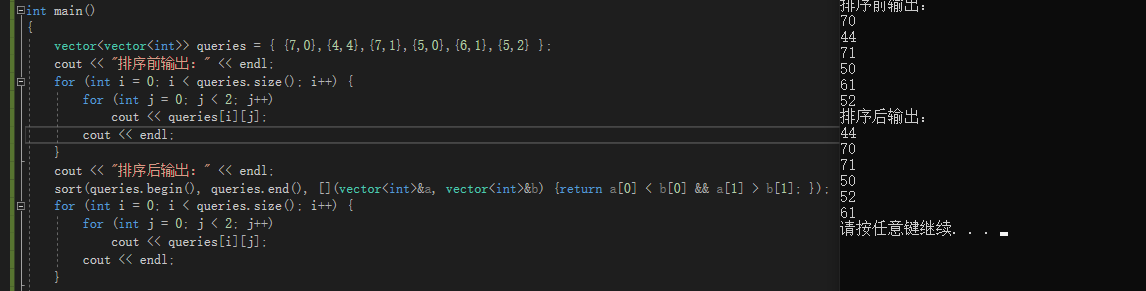正确对二维数组分别排序写法如下，只有在第一维相等的情况下再对第二维进行排序：

#include<bits/stdc++.h>
using namespace std;

int main()
{
vector<vector<int>> queries = { {7,0},{4,4},{7,1},{5,0},{6,1},{5,2} };
cout << "排序前输出：" << endl;
for (int i = 0; i < queries.size(); i++) {
for (int j = 0; j < 2; j++)
cout << queries[i][j];
cout << endl;
}
cout << "排序后输出：" << endl;
sort(queries.begin(), queries.end(), [](const vector<int>&a, const vector<int>&b) {
if(a != b) return a < b;
else            return a > b;});
for (int i = 0; i < queries.size(); i++) {
for (int j = 0; j < 2; j++)
cout << queries[i][j];
cout << endl;
}
system("pause");
return 0;
}
这下结果就对了。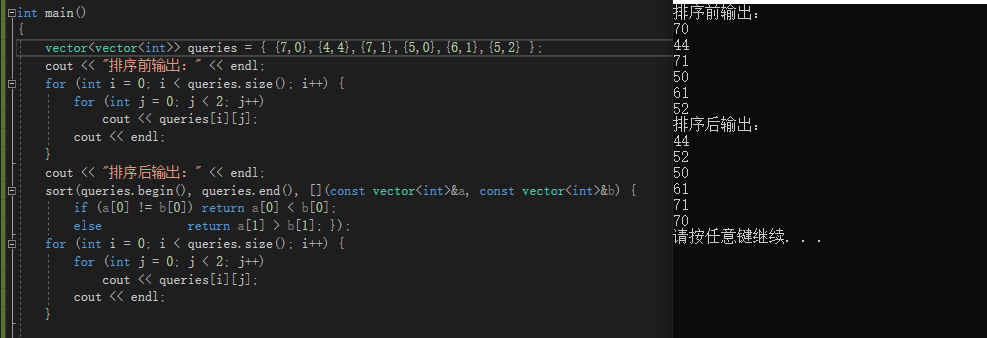展开全文贪心算法 C++ vector 排序 lamda表达式
• 不要看分数高，这个程序绝对可以对得起大家的分数。这个楼主的研究哈！反正下了评价后分数可以返还的................
• 我们知道在js中默认提供了sort函数，但是这个函数默认是按照数组内容的ascii码升序进行排列的，如果我们要对二维数组排序要如何做呢
• 二维数组中（每个一维数组的长度相同），按照如下规则排序：每一行都按照从左到右递增的顺序排序，每一列都按照从上到下递增的顺序排序 public class HashMapTest { public static void main(String[] args) { ...
二维数组中（每个一维数组的长度相同），按照如下规则排序：每一行都按照从左到右递增的顺序排序，每一列都按照从上到下递增的顺序排序
public class HashMapTest {
public static void main(String[] args) {
HashMapTest h = new HashMapTest();
int b[][] = new int[][]{{9,2,1},{4,5,5},{6,8,7},{2,5,8}};
h.rank(b);
}

/**
* 二维数组排序，从左到右，从上到下均增加
* @description TODO
* @param a
* @return
* @return int[][]
* @throws
*/
public int[][] rank(int[][] a){
int h = a.length;
int w = a.length;
System.out.println("二维数组行数为："+h+",列数为："+w);
System.out.println("二维数组排序前：");
printArray(a);
System.out.println("---------开始排序-------");

//System.out.println(result+","+result+","+result);
//最小的放在第一个位置，依次往后排
for(int x=0;x<a.length;x++){
for(int y=0;y<a[x].length;y++){
int[] result = minArray(a, x, y);
a[result][result] = a[x][y];
a[x][y]=result;
printArray(a);
System.out.println("------------");
}
}
return a;
}

//返回二维数组位置坐标和该位置后(包含)的最小的值的坐标
//x为第x行，y为第y列，且都从0开始
//result:最小值坐标的行
//result:最小值坐标的列
//result:最小值
public int[] minArray(int[][] a, int x, int y){
int[] result = new int;
int Time = 1;

int r0 = x;
int r1 = y;
//此位置后最小值
int mid = a[x][y];
//从第x行开始
for(int i=x;i<a.length;i++){
if (Time==1){
//第一次从第y列开始
//System.out.println("第x行从y列开始：");
for(int j=y;j<a[i].length;j++){
if(a[i][j]<mid){
mid = a[i][j];
r0 = i;
r1 = j;
//System.out.print(a[i][j]+",");
}
}
Time--;
}else{
//后面的从第0列开始
for(int j=0;j<a[i].length;j++){
if(a[i][j]<mid){
mid = a[i][j];
r0 = i;
r1 = j;
//System.out.print(a[i][j]+",");
}
}
}
}
result=r0;
result=r1;
result=mid;
return result;

}
//打印二维数组
public void printArray(int[][] a){
for(int i=0;i<a.length;i++){
for(int j=0;j<a[i].length;j++){
System.out.print(a[i][j]+",");
}
System.out.println("");
}
}

展开全文Java 数组排序
• 假设我们要对二维数组int[][] intervals里的一维数组按照每个一维的数组下标为 i 的元素进行升序 / 降序排序 int[][] intervals; Arrays.sort(intervals, new Comparator<int[]>() { @Override public int ...
调用API

假设我们要对二维数组int[][] intervals里的一维数组按照每个一维的数组下标为 i 的元素进行升序 / 降序排序

int[][] intervals;
Arrays.sort(intervals, new Comparator<int[]>() {
@Override
public int compare(int[] interval1, int[] interval2) {
return interval1 - interval2;
}
});


注：
Comparator<> 里的泛型要写int[], 因为我们是对每个一维数组进行排序的！int[] interval1指的是二维数组里前一个位置的一维数组int[] interval2 指的是二维数组里后一个位置的一维数组然后我们要按一维数组的首元素进行排序，所以是interval1 和 interval2! ! !升序的话就是 interval1 - interval2 ； 降序就interval2 - interval1 ！如果是按每个一维的数组下标为 i 的元素，就把 0 改成 i（注：i是具体的数字1，2，3。。)

源码分析

注：我们的目的是对二维数组中的一维数组按照每个一维数组首元素大小进行升序/降序排序

int[][] intervals;
Arrays.sort(intervals, new Comparator<int[]>(){
@Override
public int compare(int[] o1, int[] o2) {
return 0;
}
});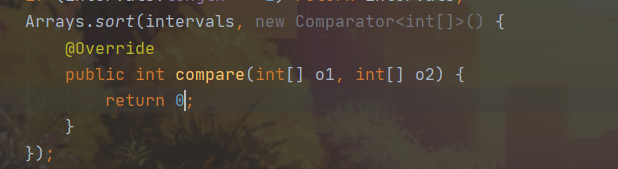按住Ctrl+A点击sort方法，查看源码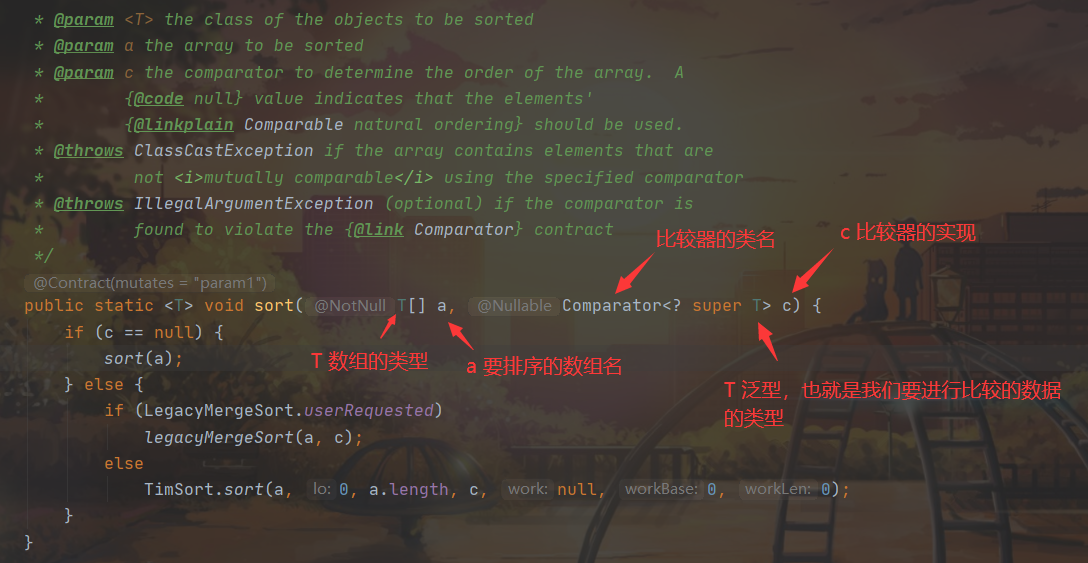（注： 这里T[] a, 在我们调用传入int[][]类型的intervals时，T就相当于int[]）

那么，这个c到底是怎么实现的呢？我们再点到legacyMergeSort()方法里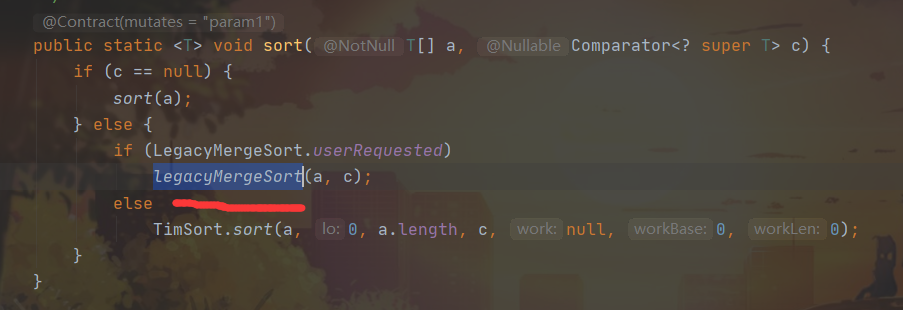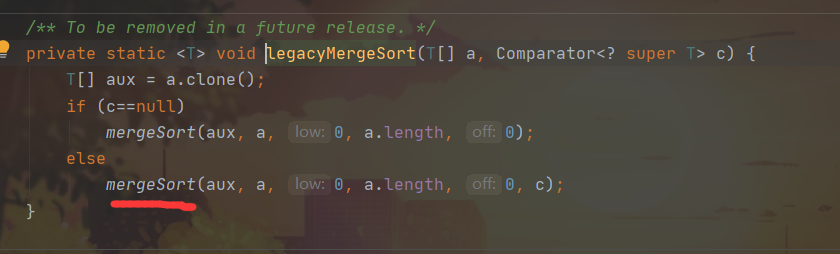再点到mergeSort()方法里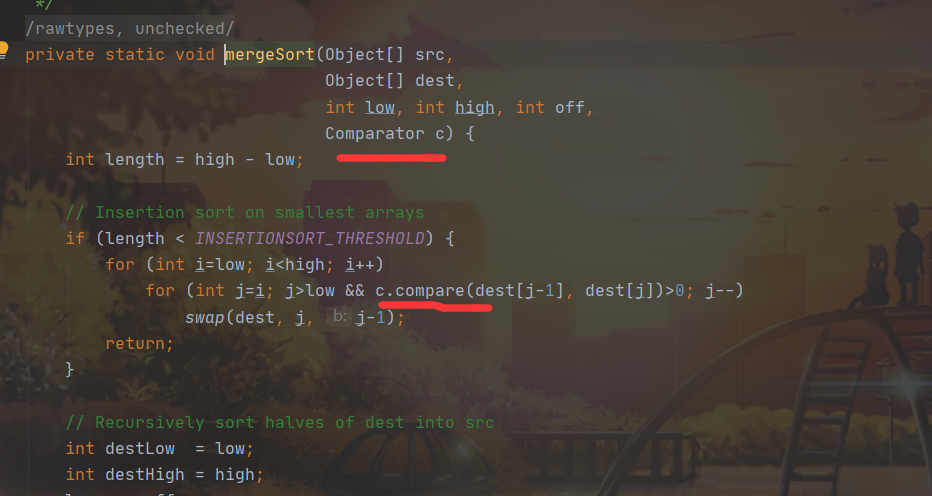这里可以很明显的看到，调用了Comparator对象c的某个方法，叫compare(), 所以我们再点到compare()里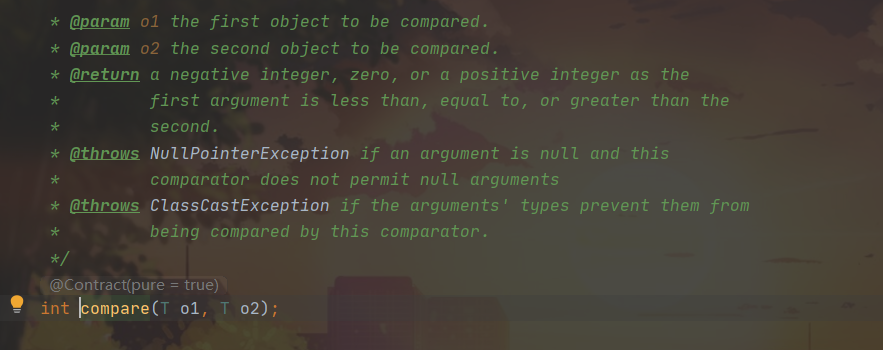可以发现，compare()方法是接口Comparator里的一个方法，所以我们在调用sort()方法时，要new 一个匿名内部类，并且重写里面的compare()方法! 而c就指向我们new出来的类！ 就像这样那么，这个compare()方法到底是干什么的呢？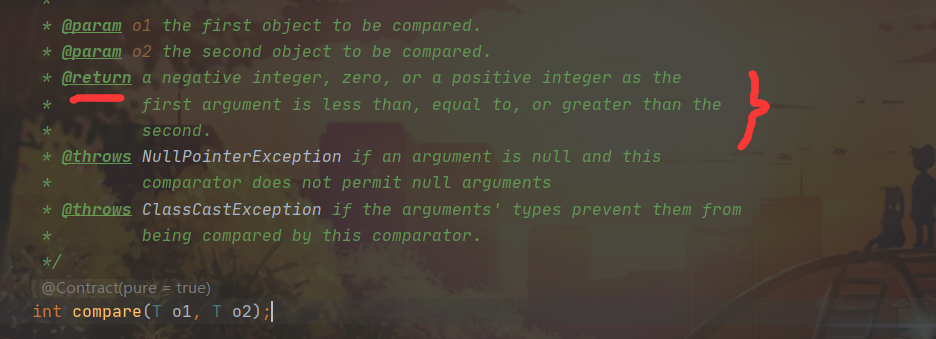根据官方的描述，我们可以知道： 如果o1 < o2 , 就返回负数 如果o1 = o2 , 就返回0 如果o1 > o2 , 就返回正数 当然，其实具体什么条件下返回正负或0 还是得看我们重写方法时怎么定义的！

那么，知道返回结果又能如何呢？我们再回到mergeSort()里！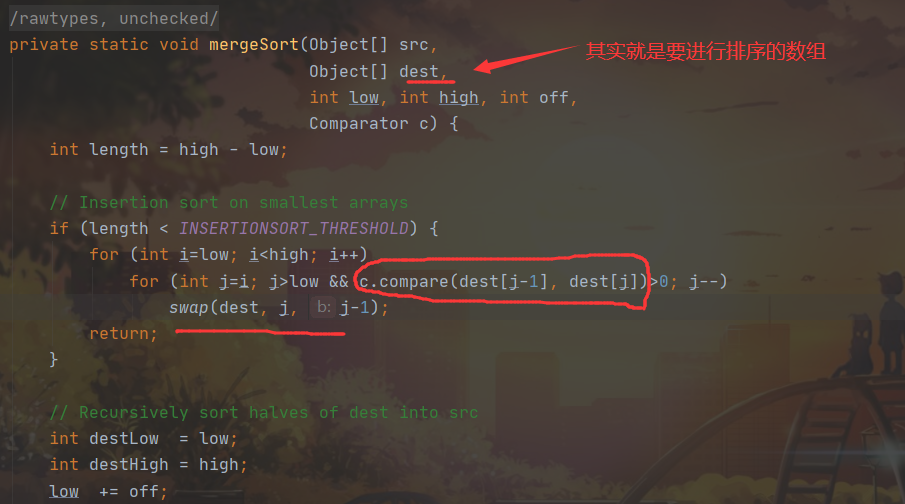可以看到，这里是说compare()返回的值 > 0并且满足一些条件的话就调用swap()方法，也就是交换（顾名思义）dest[j-1] 和 dest[j] 的位置！ 就是在compare()的返回值>0 的情况下交换dest[j-1] 和 dest[j] 的位置!

因此，对应到我们的目的:
把o1,o2改成intervals1和intervals2，方便读代码；compare()方法体里的升序逻辑就是interval1 - interval2; （如果要降序的话，就是interval2 - interval1，因为这时只有 dest[j-1] < dest[j] 了才会返回为正数）
所以，代码就是这样的了
Arrays.sort(intervals, new Comparator<int[]>() {
@Override
public int compare(int[] interval1, int[] interval2) {
return interval1 - interval2;
}
});


到了这里，可能会有人好奇，为什么compare()里的操作就是对一维数组里的元素进行操作的呢？

其实，是在调用compare()的时候，传入的是dest[j-1] 和 dest[j]，也就相当于是intervals[j-1] 和 intervals[j]， 所以我们在compare()里进行操作的时候，interval1 就相当于 intervals[j-1]，interval2 就相当于 intervals[j] （注: Java里二维数组[m][n]就相当于m 个一维数组，每个一维数组里是n个元素的一维数组）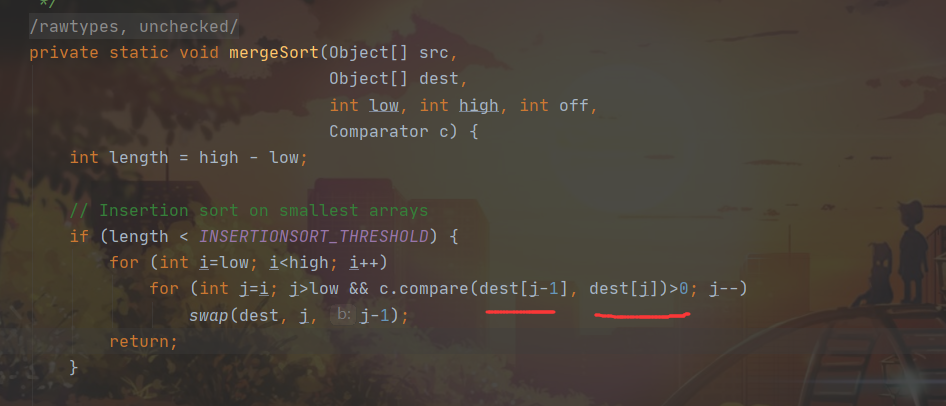展开全文• 编写一个方法public static int[][] dyadicArraySort(int a[][])，能够实现对一个二维数组a进行升序排列，要求整个二维数组中最小的值为a，然后依次是a，a….，a，a…..，使用一...java 编程 eclipse
• 二维数组，每行、列升序排列，判断某整数是否在数组中 原题如下 在一个二维数组中，每一行都按照从左到右递增的顺序排序，每一列都按照从上到下递增的顺序排序。请完成一个函数，输入这样的一个二维数组和一个整数...
• 代码： arr表示要排序的数组。 int[][] arr = new int[n][]; Arrays.sort(arr, new Comparator[]>() { @Override ... 上面代码是升序排序，当方法里的代码换成下面的代码时，表示降序排序。 return o2 - o1;java
• 运用java 实现二维数组排序 （排序要求为‘基于第一个元素排序’组合不变） 三种排序方法分别为： 选择排序，插入排序和排列排序； //选择排序 public class Sort { public static void main(String[] args) { int...java 排序算法 算法
• 排序规则：首先按照每个一维数组第一个元素进行升序排序,若第一个元素相等,则按照第个元素进行升序排序。 Arrays.sort(a, new Comparator<int[]>() { @Override public int compare(int[] o1, int[] o2) {...排序
• ## Java二维数组排序

万次阅读 2019-06-29 15:46:12排序
• ## 二维数组的排序

千次阅读 2020-03-28 13:15:04
对于需要对二维数组按某一行按升序排列，若那一行相同，则按另一行的升序排列。 方法一：定义一个类 以下为木棍加工问题，l为木棍长度，w为木棍重量，定义一个木棍类，保存木棍的长和重量。 class Wood ...
• 首先，因为是对给定的二维数组排序 那么自然就对应Java官方库中的Arrays工具类 即：Arrays.sort(T[] a, Comparator<? super T> c) 如二维整型数组 int[][] 2darr = new int; 则有： （下面写法是按照...
• 升序排序 import numpy as np boxTypes= np.array(boxTypes) boxTypes=boxTypes[np.argsort(boxTypes[:,1])]; 降序排序 import numpy as np boxTypes= np.array(boxTypes) boxTypes=boxTypes[np.argsort(-...
• 二维数组排序 第一种 正常思维 每行按一维数组比较 每行比较结束后，比较该行末位和下一行首位 代码： # include using namespace std ; int main ( ) { int a [ 3 ] [ 3 ] = { { 9 ,...
• 二维数组指定的键名排序，首先大家想到的是array_multisort函数，关于array_multisort的用法我之前也写了一篇废话不多言，我们看个实例： <?php \$data = array( 1001 => array( 'age' => 22, 'name' => '鸠...
• 二维数组如何按照名字排序 数组sort排序深入 通过利用Array原型中的sort方法,我们可以实现对数组进行排序,但是有兼容问题,在此我们不考虑兼容到ie6,7,以下内容只作为工作中的参考,如需获得兼容写法,可以@我： 需求1...
• 今天在解决leetcode的合并区间出现了需要用到二维数组的排序， 突然间想起来自己对于这方面不是很懂，虽然自己可以实现，但是实现起来很麻烦，我想看看网上有没有人有好的想法或者API直接调用。 然后就被我发现了这...
• int res= [[5,4],[6,4],[6,7],[2,3]] Arrays.sort(res, new Comparator<int[]>() { @Override public int compare...o1-o2表示对第一个元素进行升序，如果为o2-o1表示对第一个元素进行降序。 o.
• /*二维数组排序*/ public static String[][] arraySort( String array[][], int row, boolean sort,int len) { if( array!=null && len > 0 ) // 假如传入的输入不为 NULL 值 { // int len = array.length;java
• ## Python 二维数组排序

千次阅读 2018-09-25 23:56:58
多维数组的排序 之前在matlab上面想了好久。 用python实现很简单： a = [[2,3,4], [2,4,5],[3,3,4]] sorted(a, key=lambda x: (x, x)) print(a) [[2, 3, 4], [2, 4, 5], [3, 3, 4]] ...
• 排序规则: 先按数组的第一个元素进行升序排序，若第一个元素相等,则按照第个元素进行升序排序。 使用API：Arrays.sort()(T[] a, Comparator<? super T> c)，该API根据给定的比较器(设定排序方式)对指定的...java...